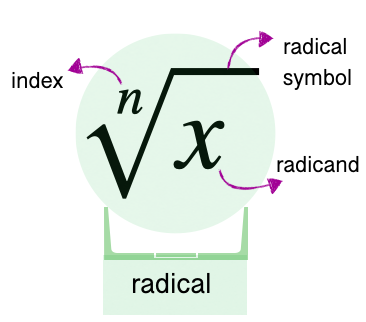407 493 6601

## Introduction

• Recall that a radical has a radicand and an index apart from the radical symbol, as shown in the figure below.• Like radicals: Radicals with the same index and radicand are called like radicals (or similar radicals). For example $\sqrt{7}$ and $5\sqrt{7}$ are a pair of like radicals.
• Unlike radicals: Radicals with different indices and radicands are called unlike radicals (or dissimilar radicals). For example $\sqrt{2}$ and $\sqrt{5}$ are a pair of unlike radicals.

• Simplify each radical term, if possible.
• Combine the like terms.
• Similar is the case with the subtraction of like radicals.

## Some Solved Examples

Question 1: Perform the indicated operation: $5x\sqrt{y}+7x\sqrt{y}-2x\sqrt{y}$

Solution: $5x\sqrt{y}+7x\sqrt{y}-2x\sqrt{y}=12x\sqrt{y}-2x\sqrt{y}=\mathbf{10}\mathbit{x}\sqrt{\mathbf{y}}$

Question 2: Perform the indicated operation:

Solution:

Question 3: Perform the indicated operation:

Solution:

Question 4: Simplify the expression: $4\sqrt{12x{y}^{4}}-y\sqrt{147x{y}^{2}}$

Solution: $4\sqrt{12x{y}^{4}}-y\sqrt{147x{y}^{2}}$$=4\sqrt{4·3x{y}^{4}}-y\sqrt{49·3x{y}^{2}}$

Question 5: Simplify the expression: $\sqrt{8x-8}+\sqrt{27x-27}-\sqrt{64x+64}$

Solution: $\sqrt{8x-8}+\sqrt{27x-27}-\sqrt{64x+64}$$=\sqrt{8\left(x-1\right)}+\sqrt{27\left(x-1\right)}-\sqrt{64\left(x+1\right)}$

Question 6: Simplify the expression: $\sqrt{{n}^{6}-{n}^{4}}-{n}^{2}\sqrt{4{n}^{2}-4}$

Solution: $\sqrt{{n}^{6}-{n}^{4}}-{n}^{2}\sqrt{4{n}^{2}-4}$$=\sqrt{{n}^{4}\left({n}^{2}-1\right)}-{n}^{2}\sqrt{4\left({n}^{2}-1\right)}$$={n}^{2}\sqrt{{n}^{2}-1}-2{n}^{2}\sqrt{{n}^{2}-1}$$=\mathbf{-}{\mathbit{n}}^{\mathbf{2}}\sqrt{{\mathbf{n}}^{\mathbf{2}}\mathbf{-}\mathbf{1}}$

Question 7: What is the simplified form of $4\sqrt{7{x}^{2}y}-5\sqrt{{x}^{2}{y}^{3}}+\sqrt{63{x}^{5}}$?

Solution: $4\sqrt{7{x}^{2}y}-5\sqrt{{x}^{2}{y}^{3}}+\sqrt{63{x}^{5}}$$=\mathbf{4}\mathbit{x}\sqrt{\mathbf{7}\mathbf{y}}\mathbf{-}\mathbf{5}\mathbit{x}\mathbit{y}\sqrt{\mathbf{y}}\mathbf{+}\mathbf{3}{\mathbit{x}}^{\mathbf{2}}\sqrt{\mathbf{7}\mathbf{x}}$

## Cheat Sheet

• Generally, we will have to simplify each term of the radical expression before we can identify the like terms.
• In addition or subtraction of radical expression, we only add or subtract the coefficients of the like terms, and the radicand part remains as it is.

## Blunder Areas

• $5\sqrt{7}+6\sqrt{7}\ne 11\sqrt{14}$ because only the coefficients of like terms are added & the radicands remain the same.
• Likewise, $8\sqrt{13x}-4\sqrt{11x}\ne 4\sqrt{2x}$.
• When simplifying and performing operations in radicals and the expression containing polynomial radicands, we always assume that the variables involved are greater than 0.
• Don't assume that expressions with unlike radicals cannot be simplified. It is possible that, after simplifying the radicals, the expression can indeed be simplified.

• Nested Radicals can be Finite or Infinite.
• Some examples of finite nested radicals are .
• Some examples of infinite nested radicals are
• The goal of simplifying these types of expression is to denest the radical.
• To denest the form $\sqrt{a±\sqrt{b}}$, the following conditions must be satisfied:
• are positive and rational.
• ${a}^{2}-b$ is a perfect square.
• The inner radical $\sqrt{b}$ must be irrational.
• If . For , the following formula will be used: $\sqrt{a+\sqrt{b}}=\sqrt{\frac{a+\sqrt{{a}^{2}-b}}{2}}+\sqrt{\frac{a-\sqrt{{a}^{2}-b}}{2}}$

• Shortcut formulas for the case of $\sqrt{a+2\sqrt{b}}$, we have: .
• One common formula for simplifying infinite nested radical is